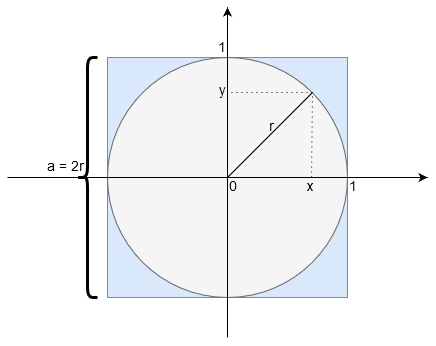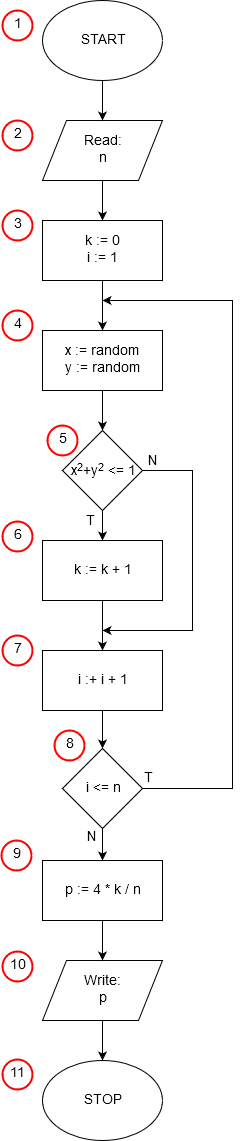# Monte Carlo Calculation of piPo = πr2

P = a2 = (2r)2 = 4r2

Po      πr2
—— = ——
P      4r2

Po      π
—— = —
P      4

Po      k
—— ≈ —
P      n

π     k
— ≈ —
4     n

4k
π
≈ ——
nImplementation of the algorithm in: Pascal, C++, Java, Python, JavaScript